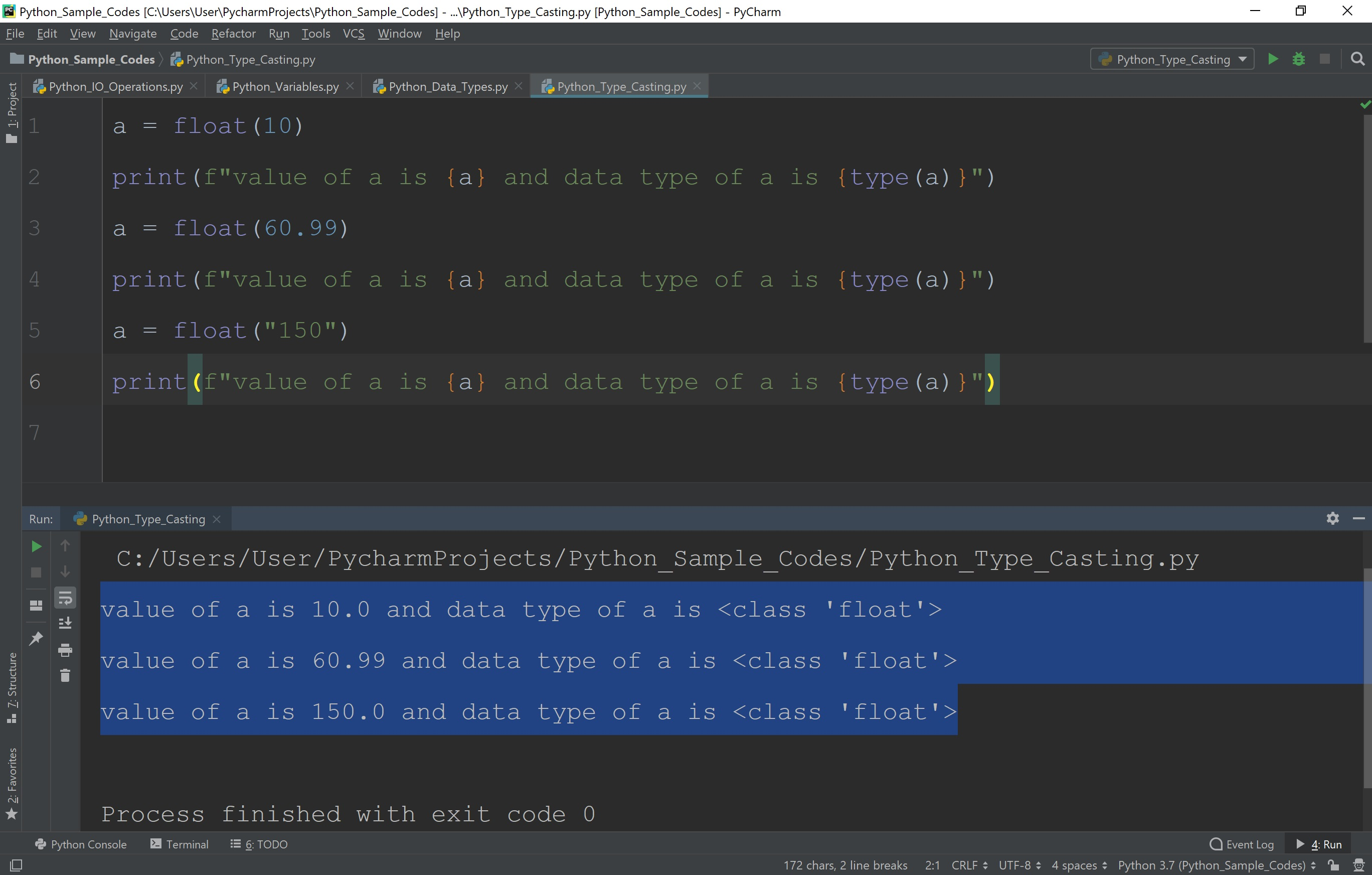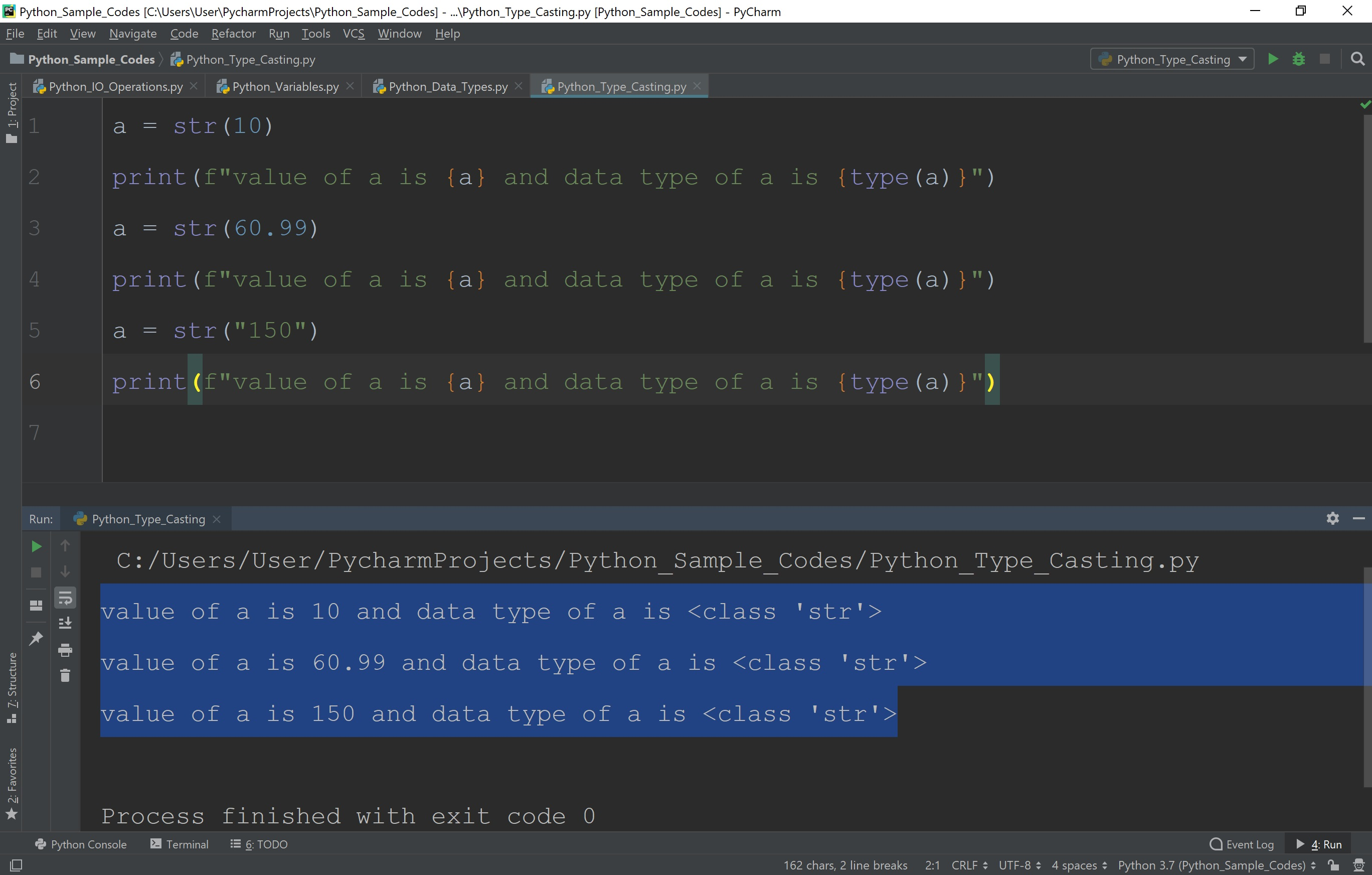The perfect place for easy learning...

×

# Python Typecasting

In all programming languages, f requently, we need to convert the values from one data type to another data type. The process of converting a value from one data type to another data type is called Typecasting or simply Casting. In Python, the typecasting is performed using built-in functions. As all the data types in Python are organized using classes, the type casting is performed using constructor functions.
The following are the constructor functions used to perform typecasting.

S.No. Function Description
1 int( ) It is used to convert an integer literal, float literal, and string literal (String must represent a whole number) to an integer value.
2 float( ) It is used to convert an integer literal, float literal, and string literal (String must represent a whole number) to a float value.
3 str( ) It is used to convert a value of any data type including strings, integer literals and float literals to a string value.

# Example - int( ) Typecasting

``````
a = int(10)
print(f"value of a is {a} and data type of a is {type(a)}")
a = int(60.99)
print(f"value of a is {a} and data type of a is {type(a)}")
a = int("150")
print(f"value of a is {a} and data type of a is {type(a)}")
```
```

When we run the above code, it produces the output as follows.# Example - float( ) Typecasting

``````
a = float(10)
print(f"value of a is {a} and data type of a is {type(a)}")
a = float(60.99)
print(f"value of a is {a} and data type of a is {type(a)}")
a = float("150")
print(f"value of a is {a} and data type of a is {type(a)}")
```
```

When we run the above code, it produces the output as follows.# Example - str( ) Typecasting

``````
a = str(10)
print(f"value of a is {a} and data type of a is {type(a)}")
a = str(60.99)
print(f"value of a is {a} and data type of a is {type(a)}")
a = str("150")
print(f"value of a is {a} and data type of a is {type(a)}")
```
```

When we run the above code, it produces the output as follows.• In Python, when an integer value is cast to float value, then it is appended with the fractional part containing zeros (0).
• In Python, when a float value s cast to an integer it rounding down to the previous whole number.